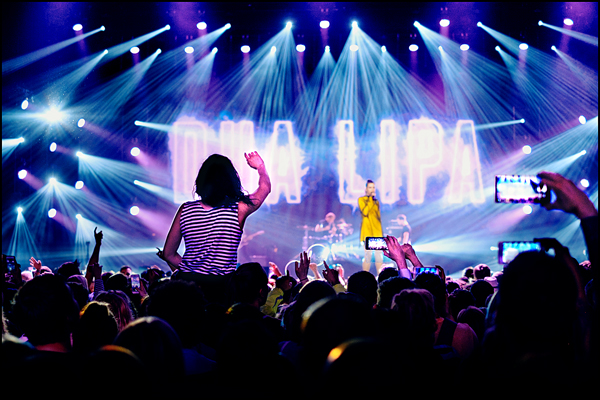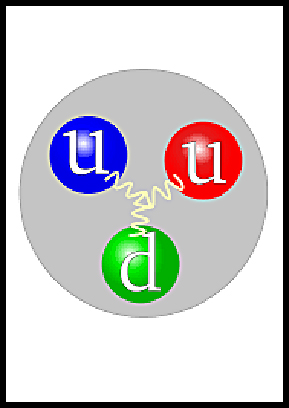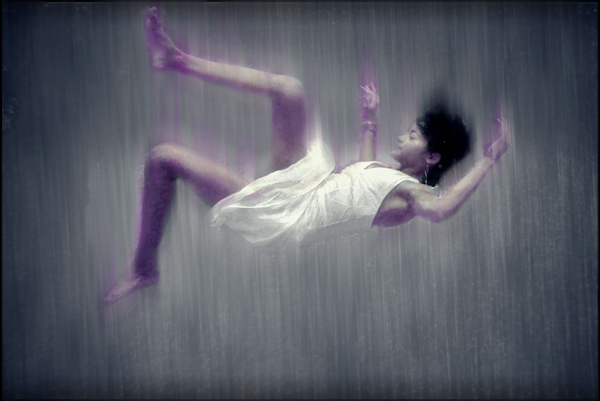# How does Energy become Mass

How does Energy become Mass

# Einstein’s famous equation E=mc^2 shows the relationship between energy and mass. According to the universal law of conservation of energy, you can’t create or destroy energy but, you can change it into a different form. Energy can change into mass and mass can turn into energy and the total amount of energy remains the same in any closed system.The energy of loud music and dance

Have you ever been told you have a lot of energy or maybe that you have a lot of weight (mass)? The law works on us also when food becomes energy for your body. Too much food and energy is stored as mass (weight). We need energy for strength and vitality required for sustained physical or mental activity.

In physics I want to examine how quantum energy moves as it changes to a mass and then to gravity. But first, what is energy made of and where does it come from.

## What is Energy

Energy is the potential or ability to do work. Work is performed when a chemical, electrical, nuclear, mechanical or other action happens due to the force of some kind of energy. That energy to do work could be from wind energy, solar energy, kinetic energy or even gravitational energy.

Today I’m discussing how energy turns into matter and how matter gets a force of gravity.

Experiments in the large hadron collider in CERN have shown that high energy photon particles have created electrons. This is a similar reaction that created electrons, quarks and gluons during the Big bang.

The reaction is from E=mc^2 where m=E/c^2.  First, energy is present and then work is done using force of the energy. In CERN the energy of kinetic energy transferred to the creation of electrons, namely energy changed into mass.

The potential energy in matter can be changed back into energy. The example is when a nuclear bomb uses matter and it’s released or changed into energy.

## What gives Matter Mass

According to Einstein’s equation the mass of particles comes from energy, m=E/c^2, which is a small number. This energy inside particles as E=mc^2, which is a large number.

The individual particles of matter are quarks, gluons and electrons. Added together the mass of these particles in a proton is a small mass. However, the mass of a proton is 99% more than the mass of the individual particles. How is this possible?

It so happens that the energy that holds particles together is responsible for 99% of the mass of matter. The strong nuclear force holds quarks and gluons together. Inside matter  the color force or Quantum Chromodynamic (QCD) force holds atoms together. The equivalence of energy to mass is what gives matter its mass. Wow.

This is an amazing thing! The energy that created the Universe during the Big bang was pure energy without mass. When the energy changed into a plasma of quarks and gluons there was still only a small amount of mass.

Because of the small mass, the creation of  the universe expanded at the speed of light.

The strong nuclear force was responsible for combining quarks and gluons together. Quarks attract more quarks and attach themselves into bundles of 3 (quarks and gluons). When the energy cooled down enough, protons and neutrons formed.

Next the particles captured electrons and stable hydrogen and helium atoms formed.

## Creation of Gravity

Maybe the first step during the creation of gravity took place when quarks and gluons joined together by the strong nuclear force. This created mass and therefore gravity due to the binding energy in the strong force. Remember energy becomes mass.

Any particle that is held together by the strong nuclear force contains quarks and gluons. Think of it as the positive pole of a magnet attracting a negative pole magnet. They are stuck together and difficult to pull apart.

If you apply enough force to separate a quark from a gluon then the energy applied changes into another quark gluon pair but not into separate quarks and gluons, They are stuck together like crazy glue.

The next step would be when quarks and gluons joined into groups of 3. The hydrogen atom has 3 quarks and 3 gluons with a rotating electron.The proton has 2 up quarks and a down quark

There are 6 different types of quarks but the only stable ones are the up quark and down quark. The other quarks decay into up and down quarks.

Quarks have a special kind of attraction to other quarks. Inside the proton the 3 quarks are playing a game of tug and war. They constantly want to capture a gluon away from the other quarks while trading it with the ones it already has.

The gluon acts like a mediator between the 3 quarks inside matter. The strong force allows the quarks to play a tugging action using energy called the color charge.

## What is Color Charge

Quarks come in 6 different flavors and 3 different energies described as a color charge. The color is only a description allowing an easy way to distinguish them.

The total color charge inside each quark must remain neutral, so that the colors of red, green and blue remains neutral or white.

This action is called quantum chromodynamics (QCD), also known as the color force.

Consider the constant action inside quarks. Quarks are the only elementary particle to experience all four fundamental forces, electromagnetism, gravity, weak and strong force.

During the color force gluons are moving around at near light speed. The constant color charge between quarks and gluons creates an excess force that could be the quantum gravity force.

This quantum force is an additive force like gravity. The power of this force is increased directly due to an increase in the mass or number of quark/gluon pairs. Since gravity is too weak between individual particle reactions no successful theory of quantum gravity exists.

## Measuring Gravity

You can calculate the gravitational force of an object by weighing the mass. But, if you know the number of protons and neutrons, the number of quark/gluon pairs gives you the gravitational force.We feel gravity every second unless we are falling with the speed of gravity.

There is no doubt that gravity is the result of the mass of an object. Inside the mass is the quantum reaction called quantum chromodynamics (QCD) which is the cause of gravity. Therefore, gravity is the combined result of the small quantum strong force and the large amount of classical mass.

The process of creating the universe with energy turned that energy into mass. Namely, photons created electrons, quarks and gluons.  That mass used the strong nuclear force to hold quarks and gluons together. The strong force created mass from energy and that mass created the color force which created the force of gravity. Like a 3 step reaction of energy creating gravity.

To Summarize:

1. The Big bang used energy to create photons

2. Photons changed into electrons, quarks and gluons

3. The strong nuclear force created protons

4. Protons captured electrons to become atoms

5. The quantum color force creates quantum gravity

6. Additive force of quantum gravity becomes classic gravity

7. Gluons and quarks are the source of gravity

8. The force of gravity depends on the speed and location of mass

9. Time dilation can be measured from the change in mass due to speed or gravity

Is this the source of gravity by combining quantum mechanics with relativity? Agree or disagree but let’s agree it looks promising.

If you found this and other articles on https://lovinthings interesting please subscribe to my blog at the bottom of this post. As always thanks for being here, and be well.

### About the Author Erik Lovin

Erik has a BSc degree and is a retired professional photographer who is now a published Author of many books. His passion is understanding how life and the universe works. He is currently blogging about the science of the Big Bang and the science of cosmology. Erik is helping his tribe with questions about the universe. His goal is to help find a theory of everything (TOE). In order to do that, he is trying to prove light has mass and that the fabric of spacetime is a false theory. We are welcoming questions and answers that you might have about the universe.